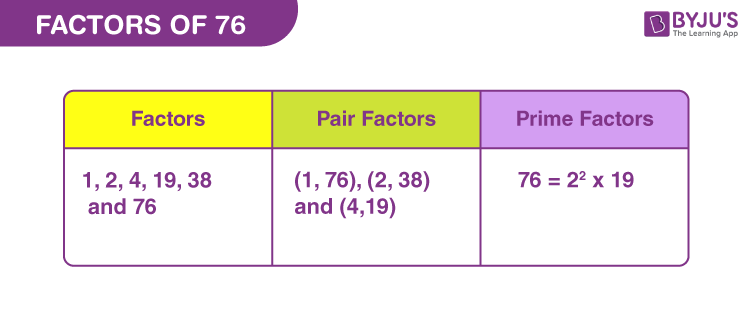# Factors of 76

Factors of 76 are the natural numbers that can divide the original number, exactly. Therefore, they are also called divisors of 76. So, if m and n are two natural numbers and the product of m and n is equal to 76, then both, m and n, are the required factors. 76 is an even number, thus divisible by 2 (by divisibility rules).

Therefore, we have figured out there are a minimum of three factors of 76 such as 1, 2 and 76. Hence, 76 is a composite number. Now let us learn the article to find more factors of 76 along with pair factors and prime factors.## How to Find the Factors of 76?

We can find the factors of 76 with simple and easy methods. One method is to find the numbers that can evenly divide 76 and another method is to find the product of two numbers that results in 76. Although we can compare both the methods simultaneously.

 Division Method Multiplication Method 76 ÷ 1 = 76 1 x 76 = 76 76 ÷ 2 = 38 2 x 38 = 76 76 ÷ 4 = 19 4 x 19 = 76 76 ÷ 19 = 4 19 x 4 = 76 76 ÷ 38 = 2 38 x 2 = 76 76 ÷ 76 = 1 76 x 1 = 76

Therefore, the factors of 76 are 1, 2, 4, 19, 38 and 76.

## Pair Factors of 76

Pair factors are determined when the product of two numbers results in the original number.

• 1 × 76 = 76
• 2 × 38 = 76
• 4 × 19 = 76

Therefore, the pair factors of 76 are (1, 76), (2, 38), and (4, 19).

If we consider the negative factors in pairs to get 76, then;

• -1 × -76 = 76
• -2 × -38 = 76
• -4 × -19 = 76

Therefore, the negative pair factors are (-1, -76), (-2, -38), and (-4, -19).

## Prime Factorisation of 76

Prime factorisation of 76 will generate the prime numbers that are the divisors of the original number. Let us see, what are the prime factors of 76.

 Dividing 76 by smallest prime number 76 ÷ 2 = 38 38 is again divisible by 2 38 ÷ 2 = 19 19 is a prime number. 19 is divisible by itself 19 ÷ 19 = 1

Therefore, the prime factors of 76 are 2 and 19.

 Prime factorisation of 76 = 2 x 2 x 19 Exponential form = 22 x 191

Also check: Prime Numbers

## Solved Examples

Q.1: Two wires are 76 cm and 38 cm long. John wants to cut the wires into pieces of equal length. What will be the maximum length of each piece of wire?

Solution: Given,

Length of wires are 76 cm and 38 cm, respectively.

To find the maximum length, we have to find the greatest common factor (GCF) of both the given values, 76 and 38. Thus, listing the factors of both the numbers, we get;

76 → 1, 2, 4, 19, 38, 76

38 → 1, 2, 19, 38

GCF of 76 and 38 is 38

Therefore, the maximum length of each piece of wire is 38 cm.

Q.2: What is the sum of all the factors of 76?

Solution: The factors of 76 are 1, 2, 4, 19, 38, and 76.

Sum = 1 + 2 + 4 + 19 + 38 + 76 = 140

Therefore, the required sum is 140

Q.3: What are the common factors of 76 and 95?

Solution: We have to list the factors of 95 and 76.

76 → 1, 2, 4, 19, 38, 76

95 → 1, 5, 19, 95

Therefore, the common factors of 76 and 95 are 1 and 19.

### Practice Questions

1. Evaluate the factors of 76 and write them in descending order.
2. Find if 4 is a factor of both 76 and 48.
3. Find the greatest number that divides 76 and 126 exactly.
4. What is the GCF of 72 and 76?

## Frequently Asked Questions on Factors of 76

### What are the factors of 76?

The factors of 76 are 1, 2, 4, 19, 38, and 76.

### What is the prime factor form of 76?

The prime factorisation of 76 is 2 x 2 x 19.

### What are the multiples of 76?

The first ten multiples of 76 are 76, 152, 228, 304, 380, 456, 532, 608, 684 and 760.

### Which is the second highest factor of 76?

The second highest factor of 76 is 38.

### Is 76 a perfect square?

76 is not a perfect square, because the square root of 76 will generate a natural number.